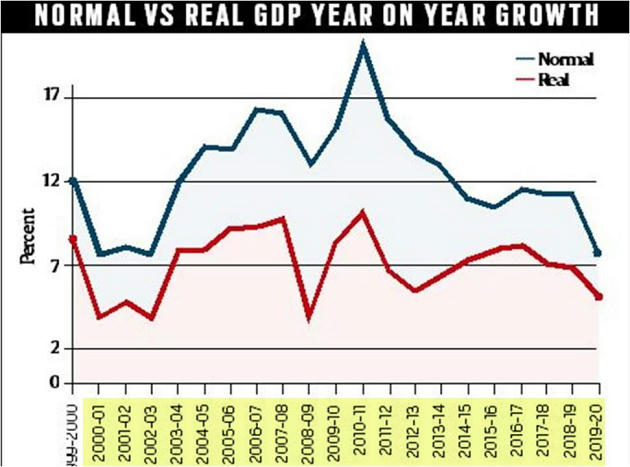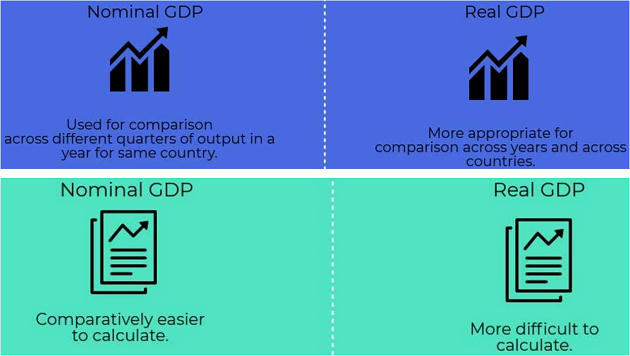#### WHAT  IS  GDP?

• GDP is the total value of all goods and services produced in the economy in  a given period of time.

## NOMINAL  GDP

• It refers to an estimate of the total value of an economy’s production and services; usually  considered over a one year period.
• It is evaluated at present market prices.

## EXAMPLE

• In 2019- We produced 40 pens & 30 books.
• If price of 1 Pen is Rs.10 and price of 1 Book is 20
• Thus, (40 × 10) + (30 ×20) = 400+600 = 1000 (It means in 2019 we added Rs.1000 to our GDP)
• Suppose till 2018,
• Our overall GDP value was 10,000. In 2019, we added Rs. 1000 to our GDP.
• Thus the GDP growth rate is 10%
• This is called Nominal GDP rate.

## REAL  GDP

• While nominal GDP is calculated at the current market price,
• Whereas real GDP adjusts for price changes due to inflation.
• Suppose in 2012, Price of Pen & Book was 5 & Rs. 10 respectively.
• In 2012, We produced same number- 40 pens & 30 books.
• Thus, (40 × 5) + (30 ×10)= 200+300= 500
• Thus, In 2012 we produced Rs.500 value of goods whereas in 2019 we produced Rs. 1000 value of goods.
• But the  important  thing  is-  we  produced  same  quantity of goods i.e. 40 pens & 30 books.

## NOMINAL  GDP  VS  REAL  GDP

• Remember till 2018, Our GDP was Rs. 10,000
• Thus in 2019. Nominal GDP growth rate will be @ 10% (current market price- Rs.1000)
• Real GDP growth rate will be @ 5% (2012 price- Rs.500)